# Flocculation cohesive sediments

Because the information on flocculation of cohesive sediments is dispersed over several articles, the most relevant sections are brought together here. They are imported from the articles Dynamics of mud transport, Sediment deposition and erosion processes and Coastal and marine sediments.

## Flocculation of cohesive sediments

A key feature of mud particles is their cohesive nature that distinguishes them from non-cohesive solid particles such as sand. In soil, only clay minerals possess electrochemical cohesion. The median diameter of the size distribution of clay minerals is typically in the range of 1-2 μm and the spread is about 0.1 to 10 μm. Since clay minerals have a large surface area relative to their weight, the electrical surface charges ensure that they easily bind to one another (coagulate) and to other substances in an aquatic environment as the result of the van der Waals forces. This process is enhanced by slimes (EPS) and mucus produced by micro-benthos and bacteria (that feed on decaying organic matter). Clays are therefore classified as cohesive sediments. Mud floc dynamics are schematically illustrated in Fig. 1.

The mean threshold diameter (grainsize) $d_T$ of about 10 μm distinguishing the fractions cohesive particles and larger cohesionless particles has been deduced from the experiments of Migniot on the settling of twenty-seven fine-grained sediments (natural and clay minerals) and a compilation of data by McCave et al. on the erosion of beds of kaolinite flocs and fine-grained quartz (< 62.5 μm) particles.

The size, structure and density of flocs are determined by the forces experienced by the aggregate-particles. These forces comprise: hydrodynamic forces (especially shear), collisions between particles and electrochemical forces (determined by the composition of solid particles and dissolved ions in the ambient water). The latter explains also why mud particles in fresh and saline water have a different structure.

Flocs contain multiple mineral particles of generally much smaller diameters (van Olphen). They are watery with buoyant weights much lower than that of the mineral, which typically has a wet bulk density in the vicinity of 2,650 kg m-3. Flocs larger than about 150 μm are notionally called macroflocs and those smaller microflocs (e.g. Eisma). Natural flocs almost always contain organic detritus in variable proportions from practically nil to 100% by weight. Their light weight and the biochemical bonding they provide can substantially influence floc properties (e.g. Gerbersdorf et al. ).

The basic building blocs of flocs are the primary particles and/or flocculi (compact aggregates, O(10 µm), which rarely break down into primary particles), which form micro-flocs (typically silt size), macro-flocs (typically fine-sand size) and organic-rich mega-flocs (typically coarse-sand size, linked to seasonal biological events, such as algae-bloom). The simultaneous occurrence of micro- and macro-flocs is attributed to the tidal dynamics, and/or sometimes to the mixing of sea- and river-born aggregates.

Despite the trend to characterize the floc structure by a fractal number , the structure is not self-similar. In general, the fractal dimension decreases with increasing floc size, which implies that the floc structures becomes more and more open and its strength decreases.

The flocculation process and modelling are described in more detail in the Annex.

## Settling Velocity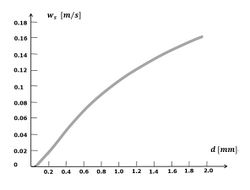Figure 2: Fall velocity of quartz spheres (specific density $s=\rho/\rho_{water}=2.65$) in still water at temperature $\theta=20^{o}C$. The fall velocity at other temperatures $\theta$ can be deduced by multiplying grainsizes $d[mm]$ by $1-0.016*(\theta-20)$.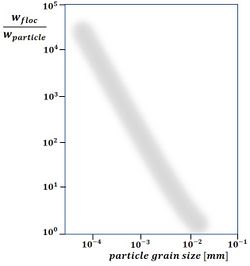Figure 3: The ratio of floc fall velocity and fall velocity of the constituent particles .

If the settling rate for very small particles, such as clay minerals, followed the curve for the settling rate of quartz spheres (see Figure 2), it would be so low that they almost never reach the bottom from suspension. The ubiquitous mud beds in coastal waters point to a different settling mechanism. This mechanism consists of flocculation, which incorporates these very small particles in flocs of much larger size and with aerodynamic shape. Observations show that flocs settle much faster than the individual constituent particles - a factor of a thousand or more, see Fig. 3. A substance that serves as a powerful binder is so-called EPS, extracellular polymeric substances . These large organic molecules (polysaccharides, proteins, nucleic acids and lipids) are exuded by living organisms and therefore omnipresent in coastal waters. Flocs grow much faster in natural seawater than in salinized distilled water. In addition to EPS, bacterial colonization also plays a role in flocculation . Flocculation is further influenced by factors such as salinity and pH of the water. When flocs grow, they not only capture smaller flocs that settle more slowly, but also other suspended sediments, such as detritus, silt and fine sand. Frequent encounters between sediment particles are important for floc growth. Flocculation is thus enhanced with a high concentration of suspended material and with a certain (low) degree of turbulence (often expressed as the turbulent shear rate $G$), dependent on the suspended sediment concentration. However, when turbulence is strong (the general case in estuaries), large flocs are broken down.

## Settling velocity formulas

Van Leussen and others introduced the following expression for the settling velocity $w_s$:

${{w}_{s}}={{w}_{sh}}(G) \Large {{\left( \frac{\phi }{{{\phi }_{h}}} \right)}^{n}} \normalsize ;\quad {{w}_{sh}}(G)={{a}_{0}} \Large \left( \frac{1+{{\lambda }_{a}}G}{1+{{\lambda }_{b}}{{G}^{2}}} \right) \normalsize ;\quad \Large \frac{\phi }{{{\phi }_{h}}} \normalsize \le 1 , \qquad (1)$

where the turbulent velocity shear rate $G$ is given by $G = \sqrt {\epsilon / \nu }=\sqrt{\Large\frac{\tau}{\rho \nu}\frac{dU}{dz}\normalsize} . \qquad (2)$

Other symbols designate: $\phi =$ the volume fraction of cohesive sediment, $\epsilon =$ the energy dissipation rate per unit mass, $\tau =$ the shear stress, $\nu =$ the kinematic viscosity, $U(z) =$ the current velocity as a function of depth $z$. The volume fraction at the onset of hindered settling is $\phi_h$ and $w_{sh}(G)$ is the maximum settling velocity for this volume fraction. The constants $a_0,\; n,\; \lambda_a, \; \lambda_b$ are independent of $G$. This expression is valid in the range $\phi \le \phi_h$. The lowest applicable value of $\phi$ can be conveniently selected to correspond to the lowest experimental concentration $C$ of suspended sediment.

Setting $G = 0$ yields $\quad {{w}_{s}}={{w}_{sh}} \Large{{\left( \frac{\phi }{{{\phi }_{h}}} \right)}^{n}} \normalsize . \qquad (3)$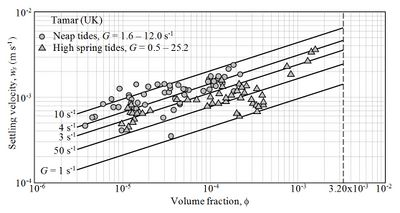Figure 4. Variation of floc settling velocity with volume fraction and shear rate. Lines are from Eq. (1); data are from Manning.

A similar formula was derived empirically by Krone with values of $w_{sh}$ and $n$ independent of $G$ deduced from settling tests on the flocs of San Francisco Bay sediment in a graduated glass cylinder. It was shown that Eq. (3) is consistent with the kinetics of inter-particle collisions and aggregation during the settling of suspended flocs previously postulated by Overbeek, with $n = 1/3$.

Returning to Eq. (1), in Fig. 4 field-collected data from a station in the Tamar estuary (UK) are included for two tidal conditions – high spring and neap (Manning ) – using an apparatus consisting of two components – LabSFLOC (Manning) and INSSEV (Manning and Dyer), which together permit optical image-based measurements of the settling velocity and the diameter as flocs fall in a column. The apparatus is taken on board the vessel and measurements are made immediately upon retrieving a sample of suspension at the desired depth below water surface. The velocity shear rate Eq. (2) is calculated from

$G= \Large [\frac{u_{*}^3}{\kappa \nu z_b}]^{0.5} \normalsize , \qquad (4)$

where $u_*$ is the known bed friction velocity, $\rho_w= 1,015 \;$ kg m-3,$\; \kappa = 0.4$ is the von Karman constant, $\nu = 1.02 \times 10^{-6} \;$ m2/s and $z_b = 0.25 \;$ m is the nominal near-bed elevation representing the sampling depth. Values of the coefficients for lines from Eq. (1) are $a_0 = 10^{-4}, \; \phi_h = 3.2 \times 10^{-3}, \; \lambda_a = 10, \; \lambda_b = 0.01, \; n = 1/3$. The $n$ value is consistent with $0.344$ obtained from data on the settling of flocs of sediment from the San Francisco Bay in laboratory flumes, even though the hydraulic conditions were not entirely comparable with those in the bay (Mehta et al. ). The value $1/3$ is also supported by data from other estuaries (Winterwerp and van Kesteren). For fixed values of $G$ (from $1$ to $50$ s-1), $w_s$ characteristically increases with $\phi$ as collisions between flocs/particles increase with particle concentration. The fifty-five data points for neap tide yield $2.34$ as the mean fractal dimension $D$ with a standard deviation $\sigma_D = 0.11$. The 50 spring tide data points give $D = 2.32$ and $\sigma_D = 0.08$. From this we may infer that variation in the tidal range did not have a significant impact on floc geometry.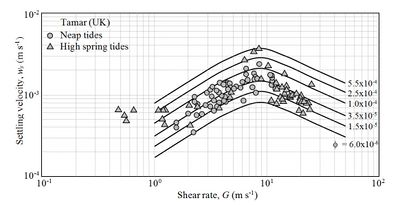Figure 5. Variation of floc settling velocity with shear rate and volume fraction. Curves are calculated from Eq. (1); data are from Manning.

In Fig. 5 the Tamar data are replotted to emphasize the role of $G$ at constant $\phi$ ranging from $3.0 \times 10^{-6}$ to $5.5 \times 10^{-4}$ consistent with measured concentrations of suspended sediment. As turbulance increases, at $G = 9.9 \approx 10 \;$ s-1 the growth and breakup processes balance and $w_s$ attains a maximum. Further increase in $G$ results in the dominance of breakup and $w_s$ decreases. Data below about $1 \;$ s-1 suggest that at these low shear rates $w_s$ is not clearly dependent on $G$. As the provenance of these low shear flocs is unknown it is reasonable to consider $1 \;$ s-1 to be the lowest value of $G$ at which Eq. (1) describes shear-induced aggregation.

Empirical formulas for the settling velocity of mud flocs have been determined by Soulsby et al. (2013) for microflocs (typical size <150 $\mu$m) and macroflocs (typical size >150 $\mu$m). Macroflocs are agglomerates of microflocs. They have the highest fall velocity, but they are less stable than the smaller microflocs. The various factors that determine the fall velocity are captured in the following empirical formulas, for macroflocs $w_{M}$ and microflocs $w_{\mu}$, respectively:

$w_{M}=\Large\frac{g B_M}{G}\normalsize \left(\Large \frac{c}{ \rho}\normalsize \right)^k \left(\Large \frac{G d_\mu^2}{\nu}\normalsize \right)^{0.33} \exp \left[-\left( \Large \frac{u_{*M}}{\sqrt{\tau / \rho}}\normalsize \right)^{0.463} \right] , \qquad w_{ \mu}= \Large \frac{g B_\mu}{G}\normalsize \left(\Large \frac{Gd^2}{\nu}\normalsize \right)^{0.78} \exp \left[-\left(\Large \frac{u_{\mu}}{\sqrt{\tau / \rho}}\normalsize \right)^{0.66} \right] , \qquad (5)$

where the index $M$ designates the macroflocs and the index $\mu$ the microflocs. The turbulent shear rate $G$ is given by Eq. (2). Other symbols stand for: $d$ the grainsize of the constituent primary particles and/or flocculi, $d_{\mu}$ the grainsize of the constituent microflocs, $\tau$ is the near-bed shear stress and $c$ the suspension concentration in $kg/l$. For the Tamar and Gironde estuaries the following parameter values were established: $B_M = 0.13, \; B_{\mu} = 0.6, \; k = 0.22, \; u_{*M} = 0.067 m/s, \; u_{*\mu} = 0.025 m/s, \; d_\mu = 10^{-4} m, \; d = 10^{-5} m$. The settling velocities observed in the Tamar and Gironde are 0.5-1 mm/s for microflocs and about 5 times larger for macroflocs.

## Hindered settling

Hindered settling, which begins when $\phi$ exceeds $\phi_h$, is manifested as a decrease in the settling velocity with increasing $\phi$. The particles are so close together that the rate at which they settle depends on the rate at which interstitial water can escape upward; this rate decreases as $\phi$ increases (and flow permeability decreases). A lutocline occurs at the depth of water at which hindered settling starts. According to Eq. (1) the maximum velocity achieved at the onset of hindered settling is dependent on the shear rate.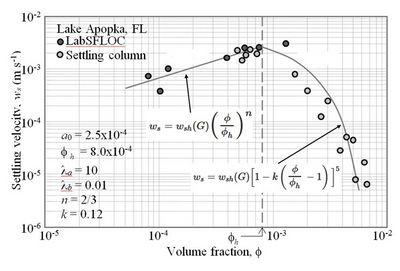Figure 6. Settling velocity as a function of volume fraction; data from Lake Apopka and curves from Eqs. (1) and (6).

The simplest model for hindered settling is the Richardson and Zaki equation

${{w}_{s}}={{w}_{sh}}(G) \Big[1-k\left( \Large \frac{\phi }{{{\phi }_{h}}} \normalsize -1 \right) \Big]^{5} \quad ; \Large \frac{\phi }{{{\phi }_{h}}} \normalsize \ge 1, \qquad (6)$

in which $k$ depends on the sediment. The exponent 5 (rounded from the experimental 4.65) was shown by the investigators to be consistent with the correction to Stokes law derived by considering the forces on a free falling particle due to neighboring particles in the viscous regime. As $w_{sh}(G)$ is not always known, it may be taken as $w_{sh}(1)$ from Eq. (1), the lowest value arising from shear-induced aggregation, i.e.

${{w}_{sh}}(1)={{a}_{0}} \Large \left( \frac{1+{{\lambda }_{a}}}{1+{{\lambda }_{b}}} \right) \normalsize . \qquad (7)$

In Lake Apopka in central Florida settling velocities of organic-rich mud ($\rho_s = 1,873 \;$ kg m-3) were measured with LabSFLOC/INSSEV and also in a laboratory settling column. As shear rates in the lake were low, assuming $G = 1 \;$ s-1, Eqs. (1) and (6) are plotted in Fig. 6 along with the data.

### Floc deposition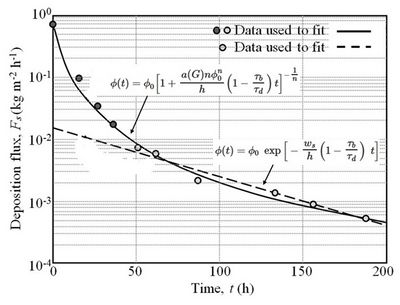Figure 7. Deposition flux of flocculated sediment from the San Francisco Bay tested in a flume (modified from Mehta et al. ).

The volume deposition flux is, according to Eq. (1),

$F_s = w_s \phi (1 - \Large\frac{\tau_b }{\tau_d}\normalsize) = a(G){\phi }^{n+1} (1 - \Large\frac{\tau_b }{\tau_d}\normalsize), \qquad (8)$

where $a(G)=w_{sh}(G) \phi_h^{-1}$, the bed shear stress ${{\tau }_{b}}={{\rho }_{w}}u_{*}^{2}$ and $\tau_d$ is called the critical shear stress for deposition. Deposition can occur only when $\tau_b$ is less than $\tau_d$.

In shallow estuaries, flocs are conveyed by current over the entire water depth $h$. The deposition of flocs in terms of time-rate of decrease of $\phi$ is then given by

$h \Large \frac{d\phi }{dt} \normalsize = - F_s =- a(G) \normalsize \left( 1-\Large \frac{{{\tau }_{b}}} {{{\tau }_{d}}} \normalsize \right){{\phi }^{n+1}}, \qquad (9)$

Solving for $\phi$ yields

$\phi (t)={{\phi }_{0}}{\Big[1+\Large \frac{a(G)n\phi _{0}^{n}}{h} \normalsize \left( 1-\Large \frac{{{\tau }_{b}}}{{{\tau }_{d}}} \normalsize \right)t \Big]^{-\Large \frac{1}{n}}} \normalsize , \qquad (10)$

where $\phi_0$ is the value of $\phi$ at the start of deposition (e.g. at the beginning of a time-step in a numerical model), which causes $\phi(t)$ to decrease (Mehta et al. ).

If $w_s$ is constant, setting $n = 0$ and $a$ constant, then Eq. (9) yields

$\phi (t)=\phi _0 \; \exp\Big[-\Large \frac{w_s}{h} \normalsize \left( 1-\Large \frac{\tau_b}{\tau_d} \normalsize \right) \; t \Big], \qquad (11)$

which is commonly known as the Krone equation with deposition flux ${{F}_{s}}={{w}_{s}}\phi$.

In Fig. 7, the Krone equation Eq. (11) and the generalized Krone equation Eq. (10) are plotted along with experimental data from a deposition test run in a flume ($h = 0.3 \;$ m, $\; \tau_b = 0.032 \;$ Pa, $\; \tau_d = 0.081 \;$ Pa) using sediment from Mare Island Strait in San Francisco Bay. The difference between the two curves is due to the inclusion of aggregation in Eq. (9) with $\phi_0 = 3.73 \times 10^{-3}, \; a = 4.3, \; n = 0.73$, which produced an order of magnitude greater net flux than from Eq. (11), with $\phi_0 = 4.11 \times 10^{-4}, \; w_s = 1.44 \times 10^{-6} \;$ m s-1. Thus it is evident that, in general, aggregation must not be ignored when modeling floc deposition.

## ANNEX: Flocculation process and modelling

Since the settling velocity of the dynamically varying mud aggregate particles changes as the floc structure changes, many attempts have been made to account for flocculation. The first simple models were empirical relationship correcting the settling velocity with a factor as a function of instantaneous concentration and turbulent dissipation rate. The next generation introduced a kinetic equation describing the aggregation and break-up of flocs . Following, more elaborate population balance equation modelling has been introduced, applied to multiple floc size fractions. In order to reduce the computational effort for large scale applications, new strategies have been proposed to work with a continuous particle size distribution  and to distinguish at least two floc populations  .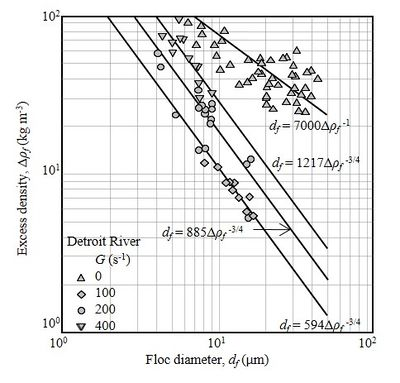Figure 8. Floc excess (bulk) density against diameter based on flocculator experiments reported in Lick.

Clay mineral particles nominally in the range of 10 to 0.1 μm are present in flocs. The floc diameter $d_f$ is determined by aggregation (processes) including floc growth and breakup. Floc growth depends on the particle-particle collision frequency which in turn is contingent upon the concentration of particles and the collision mechanism. Turbulent shear represented by the rate $G$ is the main collision mechanism in estuarine waters except during times close to slack water when collisions are promoted by flocs settling at different velocities and to a lesser extent by Brownian diffusion (Winterwerp and van Kesteren). Note that Brownian diffusion does play an important role in the aggregation of colloids, which can become large enough to be in the sedimentary size range (Mayer and Wells). Shear produces strong flocs compared to the other two mechanisms and its role is manifested as dependence of floc diameter on the Kolmogorov micro-length scale of turbulent eddies (Liggett, Fugate and Friedrichs). Floc breakup occurs when high-velocity collisions induce a stress that exceeds the shear strength of the floc, and in the absence of collisions by flow-induced shearing.

Whether the floc diameter $d_f$ increases or decreases with time $t$ is determined by the balance

$\Large \frac{d}{dt} \normalsize {{d}_{f}} = \text{rate}\ \text{of}\ \text{growth}\,\text{-}\,\text{rate}\ \text{of}\ \text{breakup}.\qquad(A1)$

Kranenburg  argued that clay flocs demonstrate fractal properties reasonably well, i.e. their structures are geometrically fairly similar, as defined by the fractal dimension $D$. Under this assumption the above equation can be represented as (Winterwerp and van Kesteren)

$\Large \frac{d}{dt} \normalsize {{d}_{f}} =\ {{k}_{g}}{{\rho }_{s}}{{\phi }^{n}}Gd_{f}^{2}-\ {{k}_{b}}{{G}^{3/2}}d_{f}^{2}({{d}_{f}}-{{d}_{p}}), \qquad (A2)$

in which $D$ is taken as 2, a typical mean value, $\phi= C/\rho_s$ is the solids volume fraction, $C$ is the sediment concentration (in units of, e.g., kg m-3), $k_g$ and $k_b$ are sediment dependent growth and breakup coefficients, respectively, and $n$ embodies the intensity of aggregation as mentioned again later. Son and Hsu proposed a modified form of Eq. (A2) by relaxing the restriction that the floc yield strength and the fractal dimension are constant.

Starting with seed mineral particles, under conditions that promote growth, $d_f$ will increase and eventually reach an equilibrium value $d_{fe}$ when the growth and breakup rates become equal. In that case $d d_f /d t = 0$ and from Eq. (A2)

${{d}_{fe}}= \Large\frac{{{k}_{g}}}{{{k}_{b}}}\frac{{{\rho }_{s}}}{\sqrt{G}} \normalsize {{\phi }^{n}}, \qquad (A3)$

where we have assumed $d_p/d_{fe} \lt \lt 1$, which is typically reasonable. This relationship indicates that $d_{fe}$ varies with ${\phi}$ as well as $G$.

The wet bulk density $\rho_f$ of flocs is usually much lower than the material density $\rho_s$. Stokes law for the settling velocity $w_s$ can be written as

${{d}_{f}}= \Large [\frac{18 \rho_w \nu {{w}_{s}}}{g\Delta {{\rho }_{f}}}]^{0.5} \normalsize , \qquad (A4)$

in which the diameter $d_{fe}$ is simply denoted as $d_f$ and $\Delta \rho_f = \rho_f - \rho_w$ is the excess floc density. From this and image-based measurements of $w_s$ and $d_f$ in a settling column $\Delta \rho_f$ can be calculated.

Very few sets of experimental data sets appear to be available in which $d_f$ is measured systematically over variable $G$ and $\phi$. As observed from the laboratory data on sediment from the Detroit River in Fig. 8, as $d_f$ increases $\Delta \rho_f$ decreases and the flocs become increasingly porous (Lick). These data were obtained in flocculators - a rotating cylinder flocculator in which the flocs grew due to shearing, and a disc flocculator in which they grew by differential settling ($G = 0$). The mean trends from the rotating flocculator can be expressed roughly as

${{d}_{f}}=\Large \frac{A(G)}{\Delta \rho _{f}^{3/4}} \normalsize , \qquad (A5)$

with $A(G)$ values given in the plot. At a constant $\Delta \rho_f$, $d_f$ increases with $G$, which means that within the range of $G = 100-400 \;$ s-1 floc growth is promoted with increasing flow shear. As a rule, at such high shear rates ($G \gt 100 \;$ s-1) breakup tends to dominate and scatters large flocs into smaller units (McAnally). This suggests that the effective shear rate in the flocculator may have been substantially lower than the value calculated from the angular velocity of the rotating cylinder. The data from the disc flocculator indicate that large diameter flocs were produced in the absence of shear-induced aggregation. In this case the exponent of $\Delta \rho_f$ is, very approximately, $-1$ indicating a somewhat different trend than Eq. (A5).

## Related articles

Dynamics of mud transport
Sediment deposition and erosion processes
Coastal and marine sediments
Mud